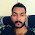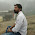C++ Program To Find The Union And Intersection Of Two Array In Increasing Order

Problem :-Write A C++ Program To Find The Union And Intersection Of Two Sorted Array In Increasing Order .

Logic :- What is Union ?.

In set theory, the union (denoted by ∪) of a collection of sets is the set of all elements in the collection. It is one of the fundamental operations through which sets can be combined and related to each other. source Wikipedia

What Is Intersection ?.

In mathematics, the intersection A ∩ B of two sets A and B is the set that contains all elements of A that also belong to B (or equivalently, all elements of B that also belong to A), but no other elements. For explanation of the symbols used in this article, refer to the table of mathematical symbols . source Wikipedia

If you have any doubt ask in comment .Till Try Below Yourself
Try This C++ Program For Draw A Perfect Christmas Tree

Solution :-

#include<iostream>
using namespace std;

void unionofarray(int a[],int b[], int m ,int n)
{
int i=0,j=0;
cout<<"\n\nUnion Of Array\n\n";

while(i<m && j<n)
{
if(a[i]<b[j])
cout<<a[i++]<<" ";
else if(a[i]>b[j])
cout<<b[j++]<<" " ;
else
{
cout<<a[i++]<<" ";
j++;
}
}
while(i<m)
cout<<a[i++]<<" ";
while(j<n)
cout<<b[j++]<<" ";
}
void intersection(int a[],int b[],int m,int n)
{
int i=0,j=0;
cout<<"\n\nIntersection Of Array\n\n";
while(i<m && j<n)
{
if(a[i]<b[j])
i++;
else if(a[i]>b[j])
j++ ;
else
{
cout<<a[i++]<<" ";
j++;
}
}
}

int main()
{
int m,i,j,n,a,b;

cout<<"Enter The Size Of First Array \n";
cin>>m;

cout<<"\nEnter The Element In First Array \n\n";

for(i=0;i<m;i++)
{
cin>>a[i];
}
cout<<"\nEnter The Size Of Second Array \n";
cin>>n;

cout<<"\nEnter The Element In Second Array \n\n";

for(j=0;j<n;j++)
{
cin>>b[j];
}

unionofarray(a,b,m,n);
intersection(a,b,m,n);

return 0;
}

Output :-

1.1.